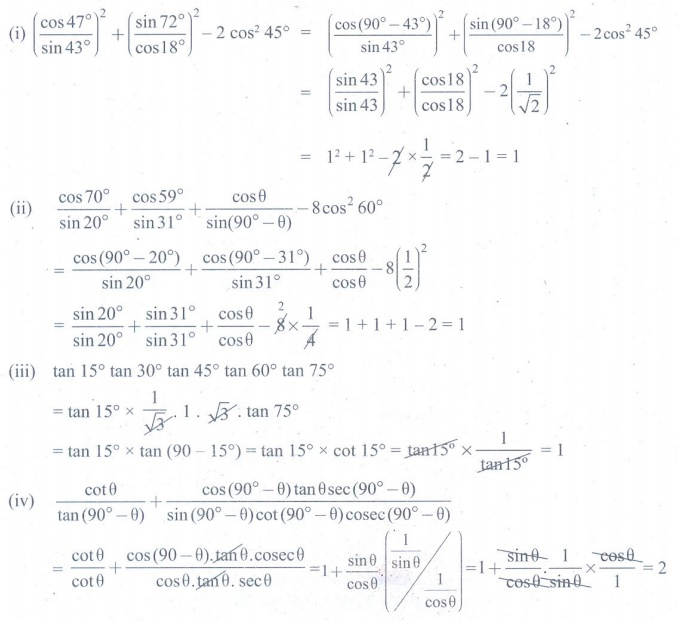Home | | Maths 9th std | Exercise 6.3: Trigonometric Ratios for Complementary Angles

# Exercise 6.3: Trigonometric Ratios for Complementary Angles

Maths : Trigonometry: Book Back, Exercise, Example Numerical Question with Answers, Solution: Exercise 6.3: Trigonometric Ratios for Complementary Angles

Trigonometric Ratios for Complementary Angles

Exercise 6.3

Find the value of the following:Thinking Corner

(i) What are the minimum and maximum values of sin θ ?

(ii) What are the minimum and maximum values of cos θ ?

Tags : Numerical Problems with Answers, Solution | Trigonometry | Maths , 9th Maths : UNIT 6 : Trigonometry
Study Material, Lecturing Notes, Assignment, Reference, Wiki description explanation, brief detail
9th Maths : UNIT 6 : Trigonometry : Exercise 6.3: Trigonometric Ratios for Complementary Angles | Numerical Problems with Answers, Solution | Trigonometry | Maths